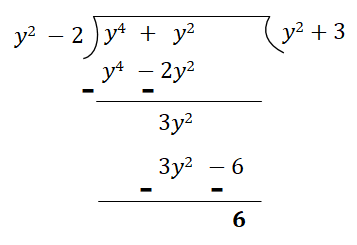# Divide:$y^{4}\ +\ y^{2}$ by $y^{2}\ -\ 2$

Given: $y^{4}\ +\ y^{2}$   and   $y^{2}\ -\ 2$.

To do: Here we have to divide $y^{4}\ +\ y^{2}$   by   $y^{2}\ -\ 2$.

Solution:

Dividing $y^{4}\ +\ y^{2}$   by   $y^{2}\ -\ 2$:So, $y^{4}\ +\ y^{2}\ =\ (y^{2}\ -\ 2)(y^{2}\ +\ 3)\ +\ 6$.

Updated on: 10-Oct-2022

27 Views